# 电势公式：电势公式的推导与电势能的公式

###### 来源：电工天下时间：2022-08-31 10:01:33 作者：老电工手机版>>

1、均匀分布的球面电荷（球面半径为r。带电量为q）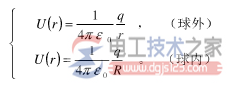2、均匀分布的球体电荷（球体的半径为r，带电量为q）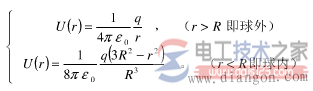3、均匀分布的无限大平面电荷（电荷面密度为sigma）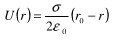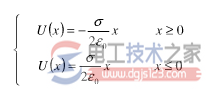4、均匀分布的无限长圆柱柱面电荷①式子：kqq*(r(n-1)-rn)/rn^2+kqq*(r(n-2)-r(n-1))/r(n-1)^2+……+kqq*(r2-r3)/r3^2+kqq*(r1-r2)/r2^2+kqq*(r0-r1)/r1^2=qu。

②式子：kqq*(r(n-1)-rn)/(rn*r(n-1))+kqq*(r(n-2)-r(n-1))/(r(n-1)*r(n-2))+……+kqq*(r2-r3)/(r3*r2)+kqq(r1-r2)/(r2*r1)+kqq*(r0-r1)/(r1*r0)=qu。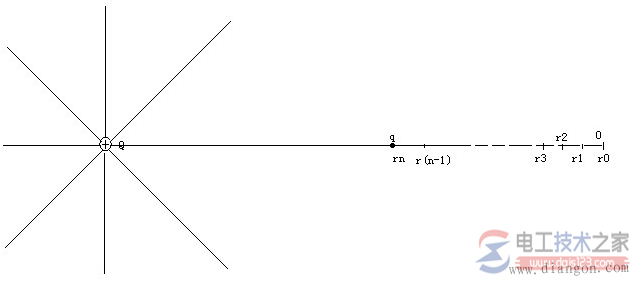`相关文章`